### Home > MC1 > Chapter 4 > Lesson 4.1.3 > Problem4-29

4-29.
1. . Complete the scaling for each number line or set of axes. Homework Help ✎

1.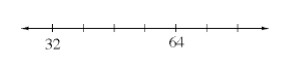2.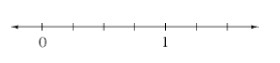3.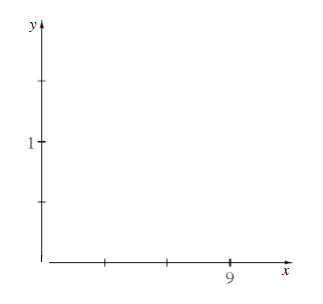4.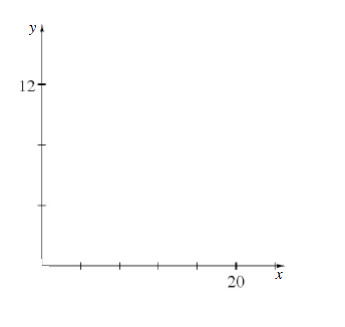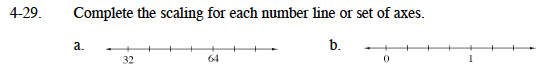Can you find the difference between 64 and 32? This number can help you find the scale of this number line.

If the difference is 32 and it is divided into 4 sections, do you know how to scale the line?

This number line should be labeled by fourths.
Can you complete the scale?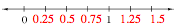To label the number line, you should have added 0.25 to each number directly before.
Does your number line look like the one above?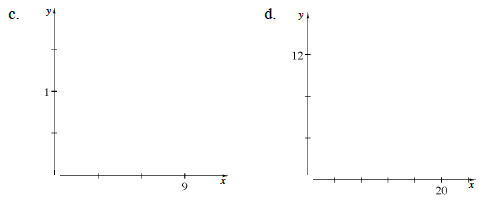Here, use the same strategies explained in parts (b) and (c). Think of each axis as a number line and complete those scales. Remember that the point where these two axes meet has a value of zero.

Try using a similar strategy as the one used in part (c). Does drawing a diagram help?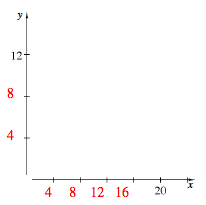Your axes should look like the ones above.